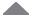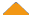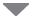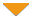Run Code  | API  | Code Wall  | Users  | Misc  | Feedback  | Login  | Theme  | Privacy  | Patreon

## Abstract Example

 run  | edit  | history  | help0What is the output of the following code: public class Q2{ public static void main (String [] args ){ int x1= 66; double x2= 33.8; x2 /= x1*4.0; System.out.println("The result of x2= "+x2); int y= 20; System.out.println("the value of y++ = " + (y++)); System.out.print("the value of --y = " + (--y)+ " "); System.out.println("the value of y = " + y); String str1= "Java programming is fun!!"; String str2= " You are TOTALLY RIGHT "; System.out.println("The length of str1 is: " + str1.length()); System.out.println("str2 in lower case: " + str2.toLowerCase()); System.out.println("The index of fun in str1 is:" + str1.indexOf("fun")); System.out.println(str2.trim()); }}   by  Amal, 4 years ago What is the output of the following code: public class Q2{ public static void main (String [] args ){ int x1= 66; double x2= 33.8; x2 /= x1*4.0; System.out.println("The result of x2= "+x2); int y= 20; System.out.println("the value of y++ = " + (y++)); System.out.print("the value of --y = " + (--y)+ " "); System.out.println("the value of y = " + y); String str1= "Java programming is fun!!"; String str2= " You are TOTALLY RIGHT "; System.out.println("The length of str1 is: " + str1.length()); System.out.println("str2 in lower case: " + str2.toLowerCase()); System.out.println("The index of fun in str1 is:" + str1.indexOf("fun")); System.out.println(str2.trim()); }}   by  Amal, 4 years ago Please log in to post a comment.